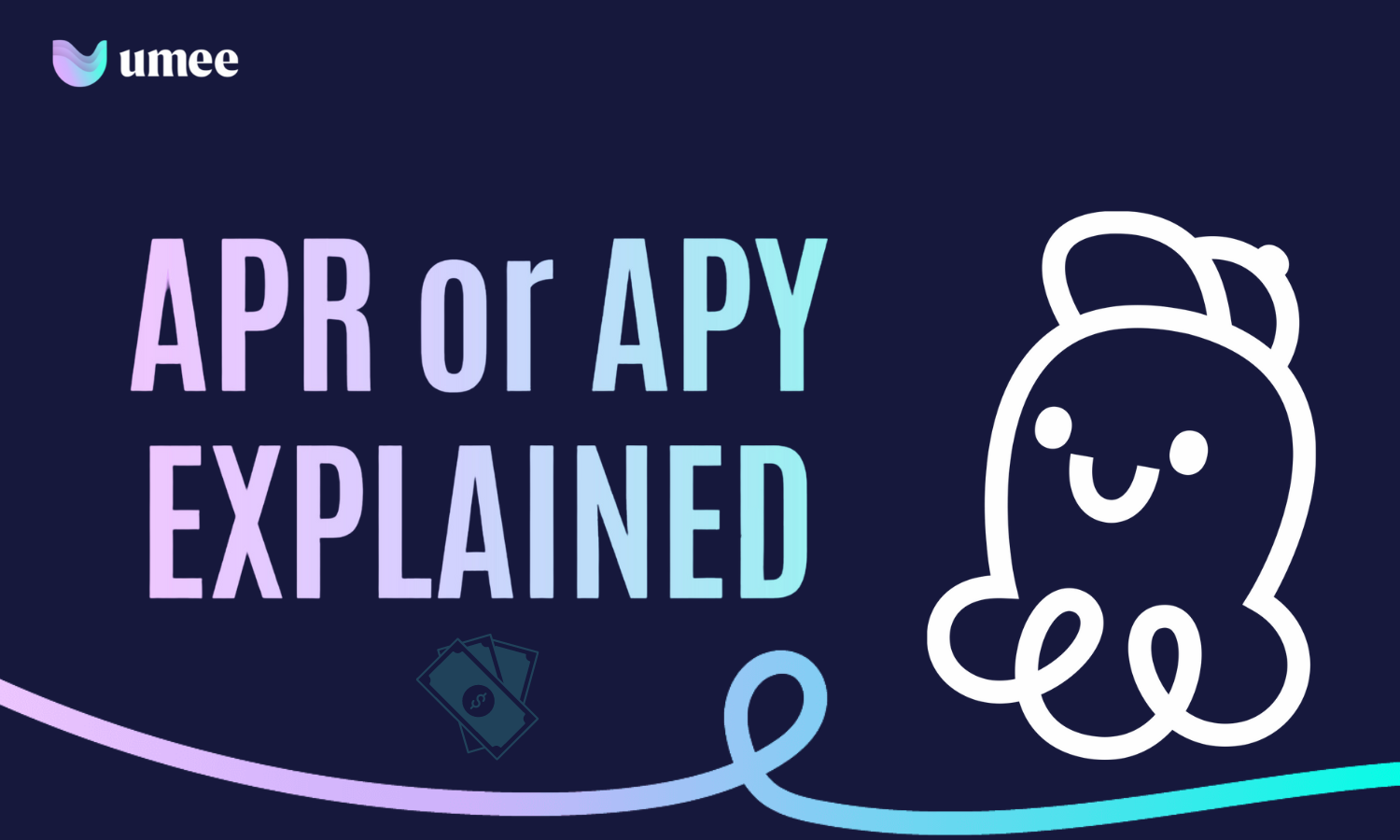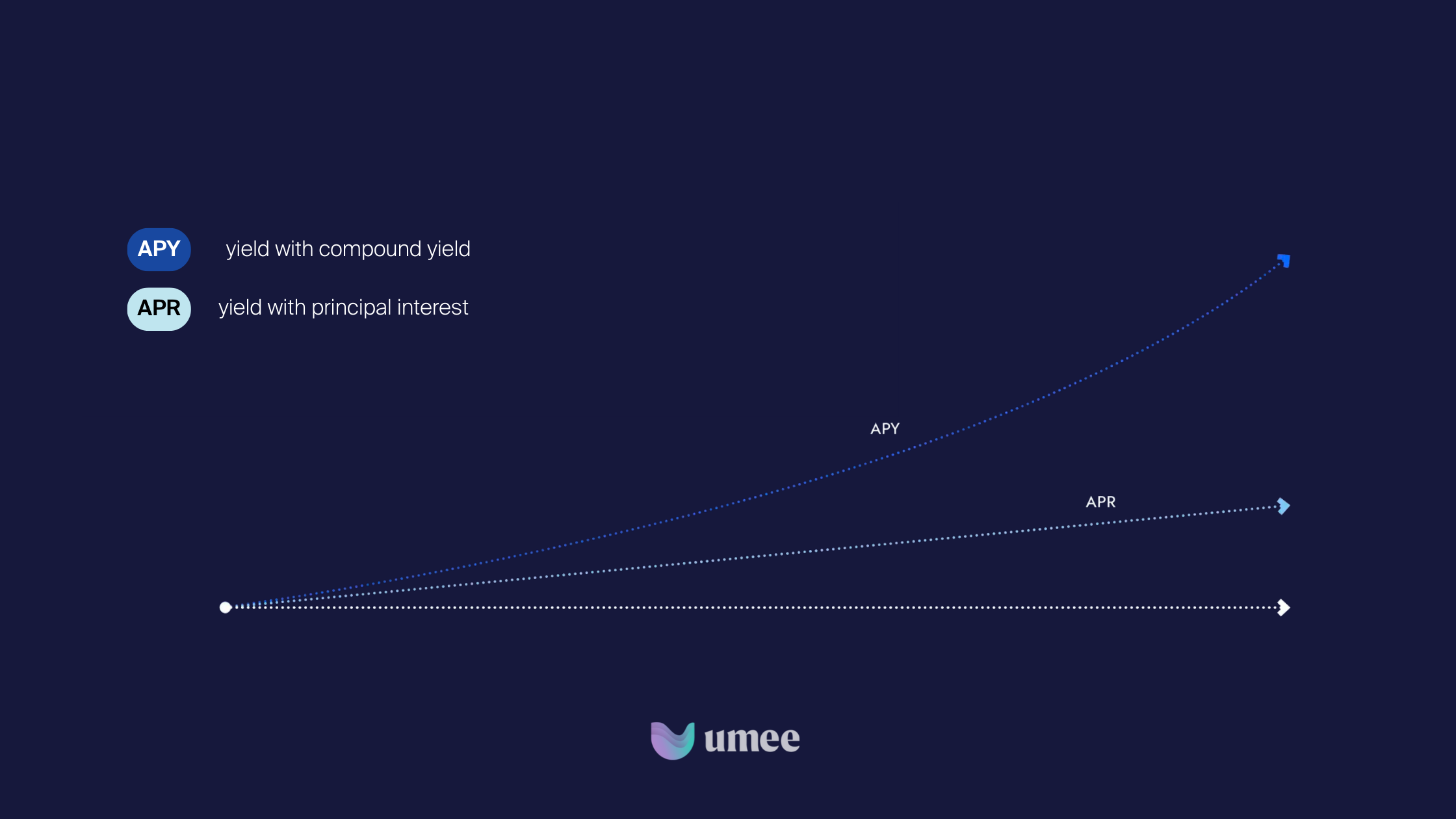May 4, 2023

# APR or APY: What's the Difference in Crypto?

In DeFi, users can earn yield on their cryptocurrencies, but it's important to understand the difference between Annual Percentage Rate (APR) and Annual Percentage Yield (APY). APR represents the yield charged or earned on an investment or loan over a year, while APY considers the compounding of yield. APY is always higher than APR because it includes the compounded yield. Understanding these terms is crucial in making informed financial decisions in DeFi.
By Naman
Share:## TLDR;

While scrolling through Crypto Twitter, you might have come across crypto platforms discussing  popular terms such as APR and APY. In DeFi, users can usually earn yield on their cryptocurrencies by interacting with DeFi applications. However, when calculating yield, two common terms are used: Annual Percentage Rate (APR) and Annual Percentage Yield (APY). Although they sound similar, they represent different calculations. In this blog post, we will explore the difference between APR and APY.

## Annual Percentage Rate (APR)

The yield charged or earned on an investment or loan over a year is called APR. It does not take into account the compounding of the yield.

The formula for calculating Annual Percentage Rate (APR) is:

APR = (Yield/Amount) x (365/Days of Repayment)

In DeFi, APR is the yield that the lender will earn on their deposited cryptocurrency over a year.

## Annual Percentage Yield (APY)

The Annual Percentage Yield (APY) considers the compounding of yield while calculating the total amount of yield earned on deposited funds over a year. It represents the true rate of return, including the yield earned on the yield.

The formula to calculate APY is:

APY = (1 + y/n)ⁿ – 1

Where "y" is the yield and "n" is the number of times the yield is compounded per year.

In DeFi, lenders earn yield on their deposited cryptocurrency, and the APY is the total amount of yield they will earn, including the compounded yield. APY is always higher than APR because it considers the compounding of yield.

## Example

For better understanding, let's use the example of a savings account in a bank. Suppose you put \$10,000 with a 10% APR. After 1 year, you'll earn \$1,000 as yield, and the total value of your investment will be \$11,000.

The yield is calculated by multiplying the principal amount and the APR. So, after 3 years, the total value of your investment will be \$13,000.However, with APY, compound yield is applied. For instance, if you put \$10,000 with 10% APY, you'll earn some yield every month. After 1 year, the final amount will be \$11,047.14. The extra \$47.14 represents the compounded yield.

## Conclusion

In conclusion, APR is a simpler and more static metric than APY. While APR comes with a fixed yield in DeFi, APY incorporates compounded yield, which varies according to the compounding frequency. A great way to remember this is that 'yield' has five letters compared to 'rate', hence APY will always be higher in comparison with APR.

While both APR and APY are important parameters in the financial ecosystem, one should not make any financial decisions solely based on them. It is essential to consider your own risk tolerance, goals, and also research the project before interacting with one. Umee strongly recommends doing your own research (DYOR) when you are navigating the DeFi world.

Share:

One weekly digest, and nothing else - promise!

Build
Developers
Tutorials
Network
Block Explorers
Validators
Testnets
Community
Socials
Governance
DAOS
Ecosystem
APPS
Support